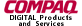gluPerspective.3glOpenGL man pages

```

```

## Name

```  gluPerspective - set up a perspective	projection matrix

```

## C Specification

```  void gluPerspective( GLdouble	fovy,
GLdouble	aspect,
GLdouble	zNear,
GLdouble	zFar )

```

## Parameters

```
fovy	  Specifies the	field of view angle, in	degrees, in the	y direction.

aspect  Specifies the	aspect ratio that determines the field of view in the
x direction.	The aspect ratio is the	ratio of x (width) to y
(height).

zNear	  Specifies the	distance from the viewer to the	near clipping plane
(always positive).

zFar	  Specifies the	distance from the viewer to the	far clipping plane
(always positive).

```

## Description

```  gluPerspective specifies a viewing frustum into the world coordinate
system.  In general, the aspect ratio	in gluPerspective should match the
aspect ratio of the associated viewport.  For	example, aspect=2.0 means the
viewer's angle of view is twice as wide in x as it is	in y. If the viewport
is twice as wide as it is tall, it displays the image	without	distortion.

The matrix generated by gluPerspective is multipled by the current matrix,
just as if glMultMatrix were called with the generated matrix.  To load the
perspective matrix onto the current matrix stack instead, precede the	call
to gluPerspective with a call	to glLoadIdentity.

Given	f defined as follows:
fovy
f	= cotangent(----)
2
The generated	matrix is

|    f				   |
|  ------  0      0            0         |
|  aspect   	    		 	   |
|					   |
|    0     f	    0		 0	   |
|					   |
|		zFar+zNear  2*zFar*zNear   |
|    0     0  ----------  ------------   |
|         	zNear-zFar   zNear-zFar	   |
|					   |
|    0     0	    -1		 0         |

```

## Notes

```  Depth	buffer precision is affected by	the values specified for zNear and
zFar.	 The greater the ratio of zFar to zNear	is, the	less effective the
depth	buffer will be at distinguishing between surfaces that are near	each
other.  If
zFar
r = -----
zNear

roughly log r	bits of	depth buffer precision are lost.  Because r
2
approaches infinity as zNear approaches zero,	zNear must never be set	to
zero.

```

```  glFrustum, glLoadIdentity, glMultMatrix, gluOrtho2D